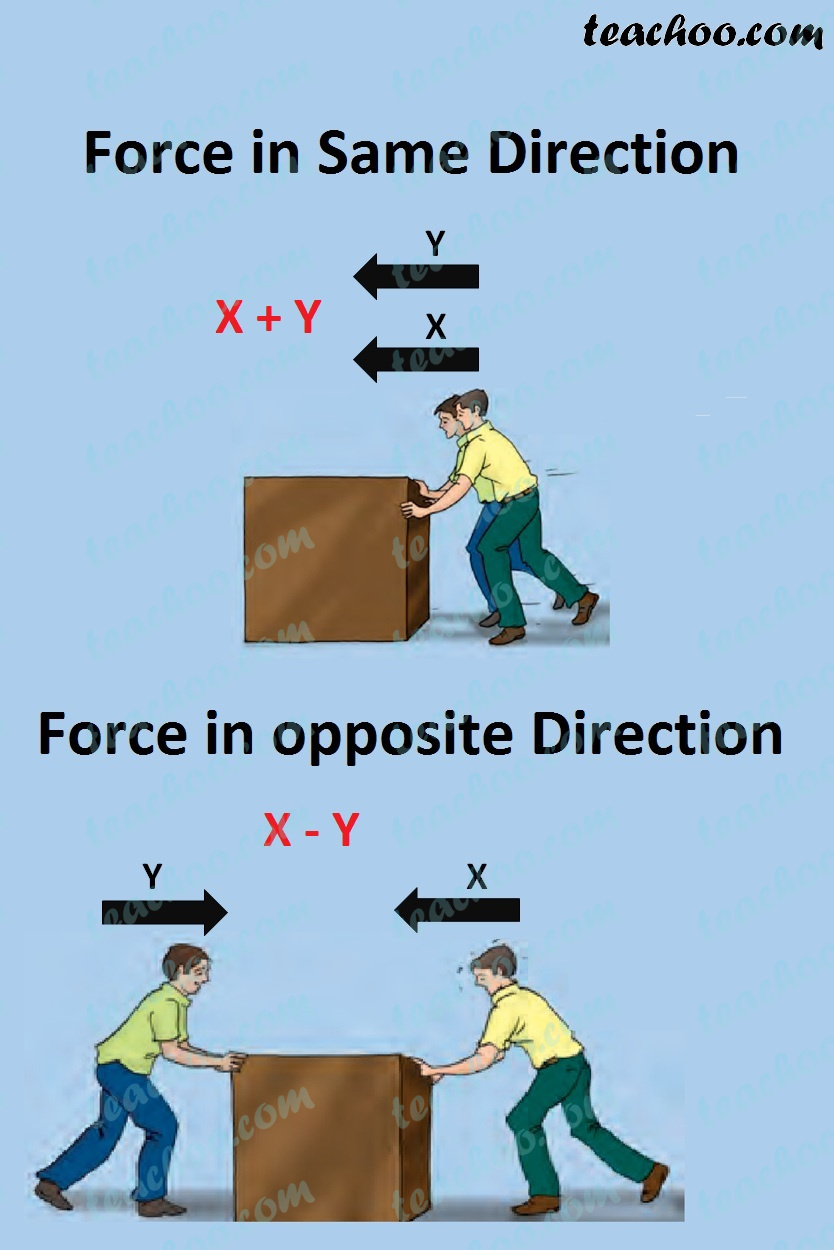Concepts

Class 8
Chapter 11 Class 8 - Force And Pressure

It means size of the force

It is sum of all forces acting on a body.

If 2 forces act in same direction,

Magnitude of force increases

It is the sum of of both forces

If 2 forces act in different direction,

Magnitude of force decreases

It is the difference of both forces

Example 1 - If 2 forces act in same direction,

Suppose 2 persons are pushing car from back

Person 1 exerts force of X

Person 2 exert force of Y

Total Force exerted on car = X + Y

Example 2 - If 2 forces act in different direction,

Magnitude of force decreases

It is the difference of both forces

Suppose 2 persons are pushing car one from back other from front

Person 1 exerts force of X

Person 2 exert force of Y

Total Force exerted on car = X - YLearn in your speed, with individual attention - Teachoo Maths 1-on-1 Class